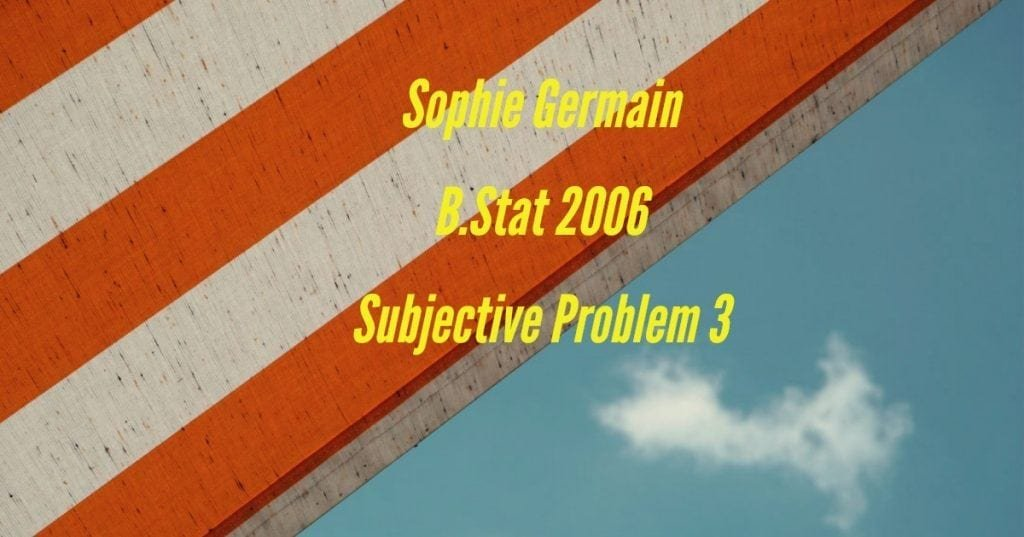How Cheenta works to ensure student success?
Explore the Back-Story

# Sophie Germain Identity | B.Stat 2006 Subjective problem 3This is a problem from the Indian Statistical Institute, ISI BStat 2006 Subjective Problem 3 based on Sophie Germain Identity. Try to solve it.

Problem:

Prove that $\mathbf{n^4 + 4^{n}}$ is composite for all values of $n$ greater than $1$.

Discussion:

Teacher: This problem uses an identity that has a fancy name: Sophie Germain identity. But what's in a name after all.

Clearly if $n$ is even the expression is composite as it is divisible by $2$. We have to check what happens when $n$ is odd.

Student: I remember Sophie Germain's identity. It says that $a^4 + 4b^4$ can be further factorized. As you hinted we can use it here.

Suppose $n = 2k +1$ (for some $k$).

So, $n^4 + 4^{2k+1} = n^4 + 4\cdot (4^k)^2 = (n^2 + 2\cdot 4^k)^2 - 2 \times n^2 \cdot 2 \cdot 4^k = (n^2 + 2 \cdot 4^k)^2 - (2 \cdot n \cdot 2^k )^2$

Thus we can use $a^2 - b^2 = (a+b)(a-b)$ identity.

So, $n^4 + 4^n = (n^2 + 2 \cdot 4^k + 2n \cdot 2^k )\times\\ (n^2 + 2 \cdot 4^k - 2n \cdot 2^k )$

Solving a few Diophantine Equations - Video

ISI 2015 BStat - BMath Objective Problems

This is a problem from the Indian Statistical Institute, ISI BStat 2006 Subjective Problem 3 based on Sophie Germain Identity. Try to solve it.

Problem:

Prove that $\mathbf{n^4 + 4^{n}}$ is composite for all values of $n$ greater than $1$.

Discussion:

Teacher: This problem uses an identity that has a fancy name: Sophie Germain identity. But what's in a name after all.

Clearly if $n$ is even the expression is composite as it is divisible by $2$. We have to check what happens when $n$ is odd.

Student: I remember Sophie Germain's identity. It says that $a^4 + 4b^4$ can be further factorized. As you hinted we can use it here.

Suppose $n = 2k +1$ (for some $k$).

So, $n^4 + 4^{2k+1} = n^4 + 4\cdot (4^k)^2 = (n^2 + 2\cdot 4^k)^2 - 2 \times n^2 \cdot 2 \cdot 4^k = (n^2 + 2 \cdot 4^k)^2 - (2 \cdot n \cdot 2^k )^2$

Thus we can use $a^2 - b^2 = (a+b)(a-b)$ identity.

So, $n^4 + 4^n = (n^2 + 2 \cdot 4^k + 2n \cdot 2^k )\times\\ (n^2 + 2 \cdot 4^k - 2n \cdot 2^k )$

Solving a few Diophantine Equations - Video

ISI 2015 BStat - BMath Objective Problems

This site uses Akismet to reduce spam. Learn how your comment data is processed.

### One comment on “Sophie Germain Identity | B.Stat 2006 Subjective problem 3”

1. […] Sophie Germain (B.Stat 2006, subjective problem 3) […]

### Knowledge Partner